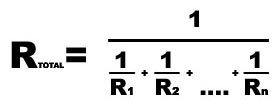# Parallel Resistor, Inductor or series capacitor calculator

This calculator can determine the resistance of up to 10 resistors or inductors in parallel or capacitors in series. Enter values into the boxes below and click CALCULATE. The result will appear in the box below that button.As a test, if you input resistances of 8, 15 and 22 ohms, your answer should be 4.2173 ohms.
Clicking the RESET button will clear all the boxes.

This calculator can solve other math problems.
Calculating resistors in parallel is PRECISELY the same as the calculations required for INDUCTORS in PARALLEL or for CAPACITORS in SERIES.

This calculator can be used for work problems. For example, ‘A’ can paint a room in 5 hours and ‘B’ can paint a room in 6 hours. If they both work together how long will the job take? Input the 5 and 6 just as if they were resistors and get your answer.

This calculator can be used for work problems. For example, ‘A’ can paint a room in 5 hours and ‘B’ can paint a room in 6 hours. If they both work together how long will the job take? Input the 5 and 6 just as if they were resistors and get your answer.

Significant Figures >>>

Numbers are displayed in scientific notation with the amount of significant figures you specify. For easier readibility, numbers between .001 and 1,000 will not be in scientific notation but will still have the same precision.
You may change the number of significant figures displayed by changing the number in the box above.
Most browsers, will display the answers properly but if you are seeing no answers at all, enter a zero in the box above, which will eliminate all formatting but at least you will see the answers.